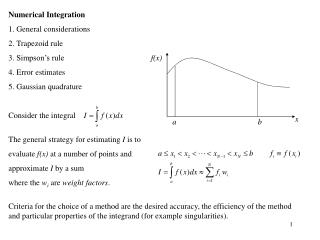DownloadDownload PresentationNumerical Integration General considerations Trapezoid rule Simpson’s rule Error estimates

# Numerical Integration General considerations Trapezoid rule Simpson’s rule Error estimates

Download Presentation## Numerical Integration General considerations Trapezoid rule Simpson’s rule Error estimates

- - - - - - - - - - - - - - - - - - - - - - - - - - - E N D - - - - - - - - - - - - - - - - - - - - - - - - - - -
##### Presentation Transcript

1. f(x) x a b • Numerical Integration • General considerations • Trapezoid rule • Simpson’s rule • Error estimates • Gaussian quadrature • Consider the integral • The general strategy for estimating I is to • evaluate f(x) at a number of points and • approximate I by a sum • where the wi are weight factors. Criteria for the choice of a method are the desired accuracy, the efficiency of the method and particular properties of the integrand (for example singularities).

2. f(x) N=5, n=4 T1 T2 T3 T4 x a=x1 x2 x3 x4 b=x5 h • Trapezoid rule • N evenly spaced points xi • n=N-1 intervals of size h • in each interval, approximate the curve f(x) by a straight line (Taylor expansion to first order)

3. f(x) N=5 x a=x1 x2 x3 x4 b=x5 • Simpson’s rule • N evenly spaced points xi • n=N-1 intervals of size h • in each pair of intervals, approximate the curve f(x) by a parabola (Taylor expansion to second order) h

4. The Simpson rule approximation IS to the integral I is called Sn in Cooper, A Matlab Companion … , pp 172-173 • The number of intervals (n = N-1) has to be even! • The sum over the weights provides a useful check of the derivation

5. Error estimates Note: the value of the prefactor for S is incorrect because we have also approximated the second derivative of f (x) when we derived Simpson’s rule. A more careful analysis yields:

6. Even though we have used second order polynomials to approximate the function over the pairs of intervals, the Simpson rule yields exact results for polynomials up to third order, since the error is proportional to a fourth-order derivative. • ES 1/n4  doubling the number of intervals reduces the error by a factor 16 . • The error estimates for the Trapezoid rule are derived in a similar way and given by Matlab offers a numerical integration routine quad that uses an adaptive Simpson quadrature. Quadruature is the general term for numerical integration methods (from counting squares under a curve). An adaptive integration method uses different interval sizes depending on the behavior of the function and the desired tolerance. Assume we want to evaluate the integral between two points u and v with au<vb: 1. Split [u,v] into two intervals of size h and use Simpson’s rule, call the result S(2)2. Split [u,v] into four intervals of size h/2 and use Simpson’s rule, call the result S(4)3. Calculate the error estimate (2) | S(4)- S(2)|4. If the error is smaller than the tolerance, accept the result and go on to the next interval [v,w]. Otherwise, split [u,v] into 8 intervals of size h/4 , etc.

7. Gaussian quadrature Please read the handouts from: A. L. Garcia, Numerical Methods for Physics, pp 325-328, and Landau and Paez, Computational Physics, pp 55-57 Remember the general form of numerical integration: Gaussian quadratures use evaluation points xi (not evenly spaced) and weights wi that yield an optimum approximation to the integral for specific types of functions. Gaussian quadrature is the preferred method if you can choose a type (Legendre, Laguerre, Chebyshev, etc. ) appropriate for your problem. In that case, you can achieve much higher accuracy with many fewer evaluation points compared to the other integration methods. However, in general, it is difficult to estimate the error and there is no guarantee that the result is a good approximation to the integral. Matlab offers a routine quadl that employs a variant of Gaussian quadrature. It may work very well, but be careful when using it and check your results with a different method from time to time.